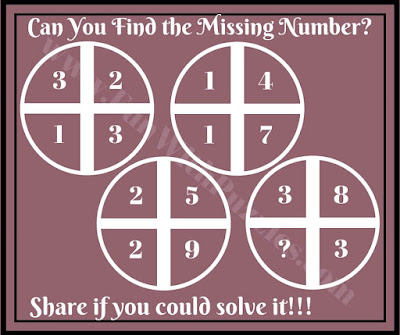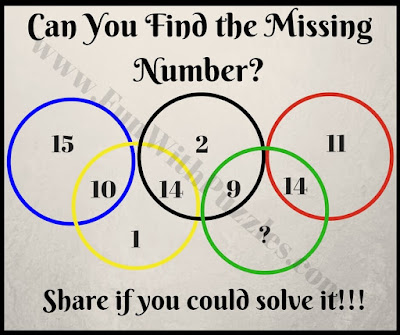## Thursday, May 28, 2020

Mathematics is a very interesting subject. I love to solve Mathematical Puzzles. I have created many different types of Maths Brain Teasers which are published under different posts on this website. Out of these Mathematical Puzzles, most famous puzzles are the ones which are created around a Geometrical shape such as Circle, Triangle, Rectangle etc. In these Maths Puzzles, numbers around these shapes are given which relates to each other with some Mathematical Pattern. Here are similar Brain Cracking Maths Puzzles which are themed around Circle.
In these Maths Circle Puzzles, some numbers are given in or around Circle. These numbers are related to each other Mathematically. Your challenge is to find the Mathematical pattern on which these numbers relates to each other and then solve for the missing number which will replace the question mark.
Answers of these Brain Cracking Maths Circle Puzzles will be given once there are few answers from our readers. Do post your answers for these puzzles in the comment section of this post.1. Can you find the missing number?2. Can you solve it for the missing number?3. Which number will replace the question mark?4. Can you find missing number which will replace the question mark?5. Solve and find the missing number.

Also, don't forget to check out similar Mathematical Puzzles as mentioned below

## List of Brain Cracking Maths Questions

1. Mathematics Logic Brain Cracking Problems with Answers and Explanations: It contains brain cracking problems of Mathematics which will not only test your Maths skills but also your Logical Reasoning.

2. Simple Math Riddles with Answers to Twist your Brain: Here is another set of easy and simple Maths brain teasers which will twist your brain.

3. Fun Brain Teasers For Kids, Teens and Adults with Answers to Challenge your Mind: These are the most liked fun brain teasers on which website which contains a mix of Maths, Logic and word brain teasers.

4. The Brain Wiring Test with Answers: After solving all these mind cracking puzzles, its time now to give brain wiring test to check your Maths, Logical as well as Observational skills.

----------------------------------------------
Here is an answer to 15th Brain Teaser Questions of the post titled "Simple Math Riddles for Teens and Kids with Answers to Twist your Brain". We have hidden this answer to avoid any spoiler. Do select the text below to read the answer.
--------------------------
The answer is 26. The is Simple series with the difference in number is 2 more than the difference in previous numbers. So difference starts with 5 and increases in the series 5 7 9 11 and 13.
--------------------------

1.1.Thank for asking for the answers to these Circle Puzzles. Solutions of these #puzzles will be published soon.

2.1.Solutions to these Circle #puzzles will be posted soon.

3.answer for 2nd question is 7.

1.Our answer to this puzzle question is different. It will be great if you can write down your logical reasoning which is used to get this answer?

A comment doesn't cost a thing. Please drop a comment below to boost the author's morale.